/

### Function Description

Returns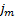, the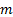’th percentile (here being between 0 and 1) of a series of observations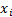(for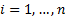) treating the lowest value as corresponding to the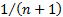’th percentile and the highest as corresponding to the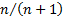’th percentile.

Different commentators use different ways of identifying percentiles (i.e. quantiles) from finite sized samples. The Nematrian website uses the following methodology (for MnPercentileExc but not for, say, MnWeightedPercentile).

First sort the series in ascending order. The’th percentile (i.e.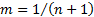) is the value ranked first in this ordering and the 100’th percentile (i.e.) is the value ranked last in this ordering. The percentile ranking given to other observations are equally spaced between within this range depending on the position of the observation in this ordered list. Other percentiles are calculated by linear interpolation between the two values straddling the given percentile rank.

The equivalent function in Microsoft Excel is PERCENTILE.EXC.

For a similar function that returns the minimum of thefor the 0’th percentile and the maximum for the 1’th percentile, i.e. corresponds to Microsoft Excel’s PERCENTILE or PERCENTILE.INC function, then see MnPercentile.

Contents | Prev | Next